# clinic-answer-eight-queens-python

Eight Queens Solver Desktop Application

Example answer to the Eight Queens Puzzle of Chapter 3 of the LinkedIn Learning: Code Clinic: Python.

Note that this is not my first wxPython 4 application, nor my first Python 3 design. The learning curve was moderate.

## Derived requirements:

• The application shall be cross-platform between Windows 10 Pro 20H2, Ubuntu 2018.04 LTS, and macOS 10.15 Catalina.
• The application shall be possible to distribute closed-source by not relying upon a copy-left graphical toolkit.
• The application shall search for all chess board permutations without using a brute-force approach.
• The application shall graphically represent the chess board with eight queen pieces.
• The application shall be capable of displaying any of the 92 puzzle solutions, one at a time.

## Refined requirements:

• The application shall use the wxWidgets 3.0.x/3.1.x graphical toolkit via wxPython 4.x for the design of the window frame and controls.
• The Anaconda3 2020.11 distribution shall be used as the Python 3 installation of choice.
• The application shall provide solutions ordered as a depth-first traversal of the chess board rows, solving the puzzle in like fashion as a human would solve on a physical board.
• Examples in the blog post shall give more than hints as this puzzle is well discussed on-line.

### Eight Queens Solver App, Python, on macOS Catalina.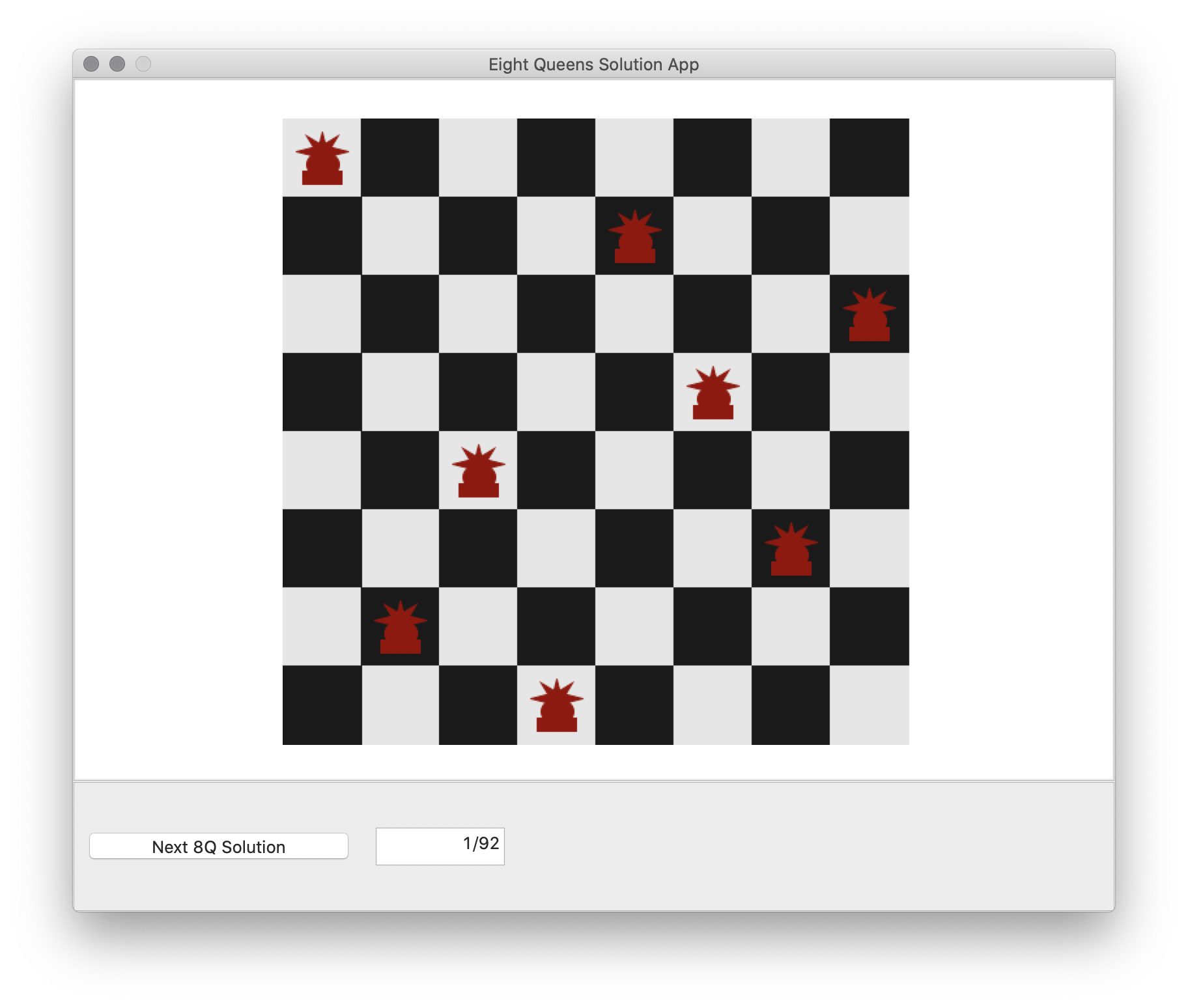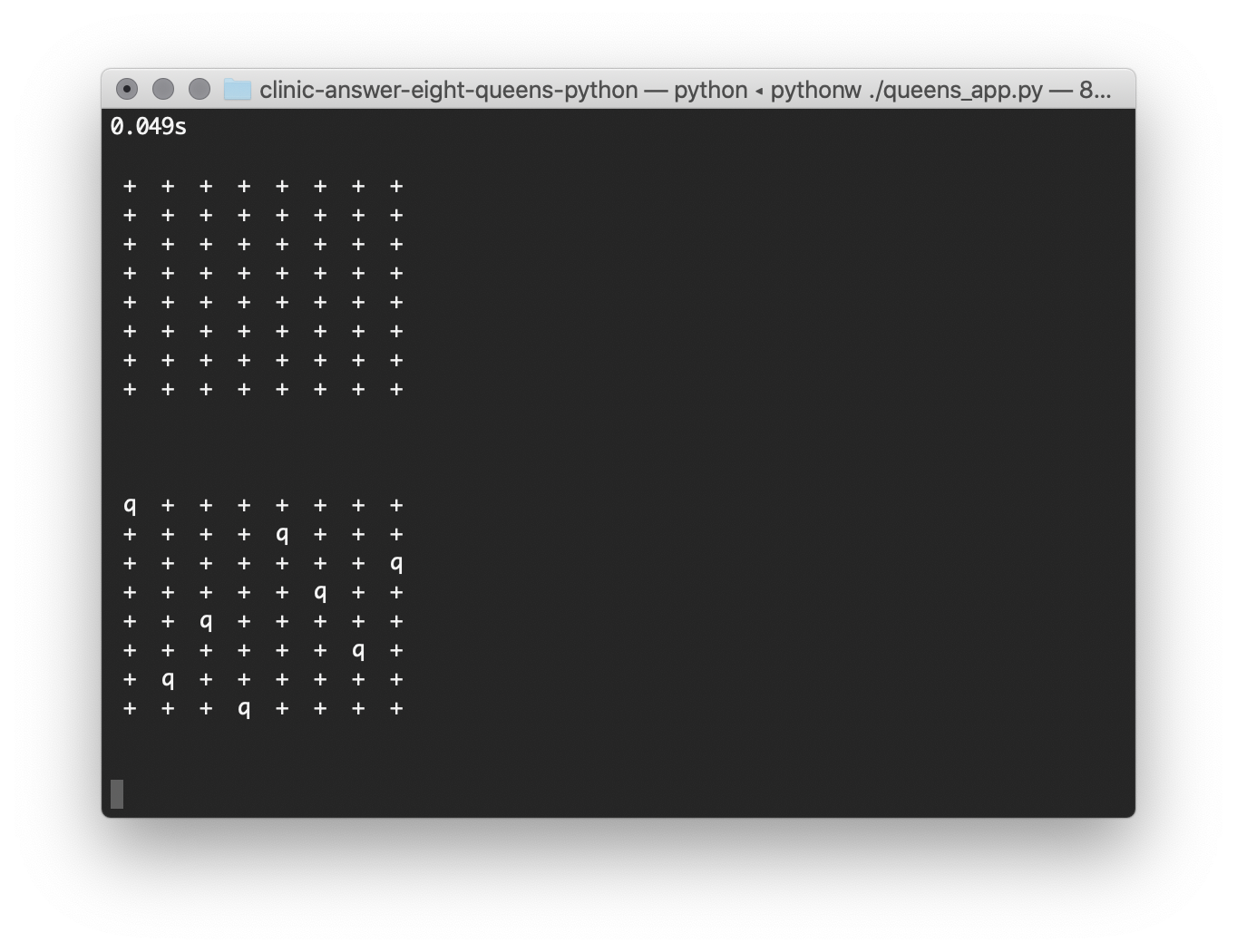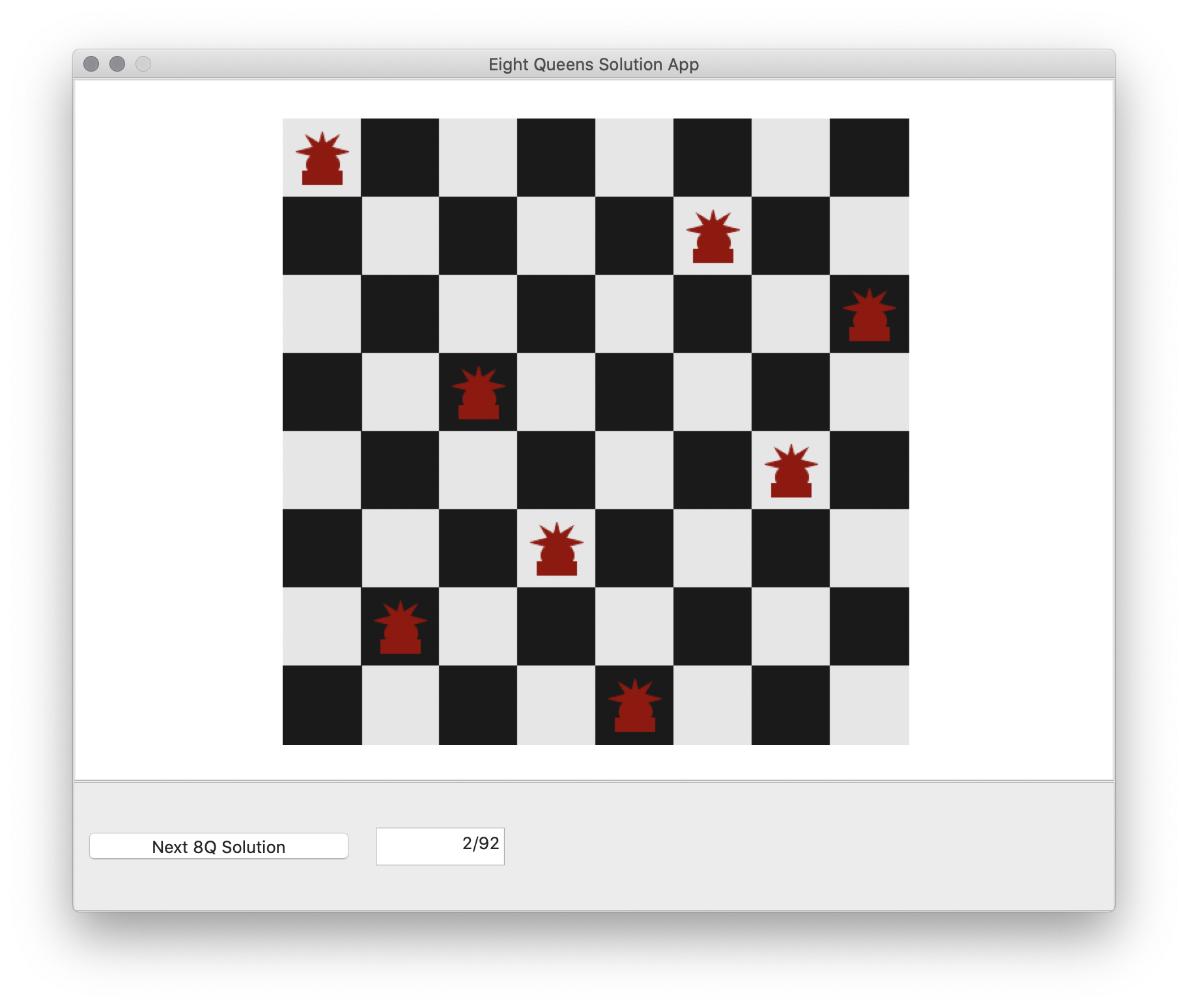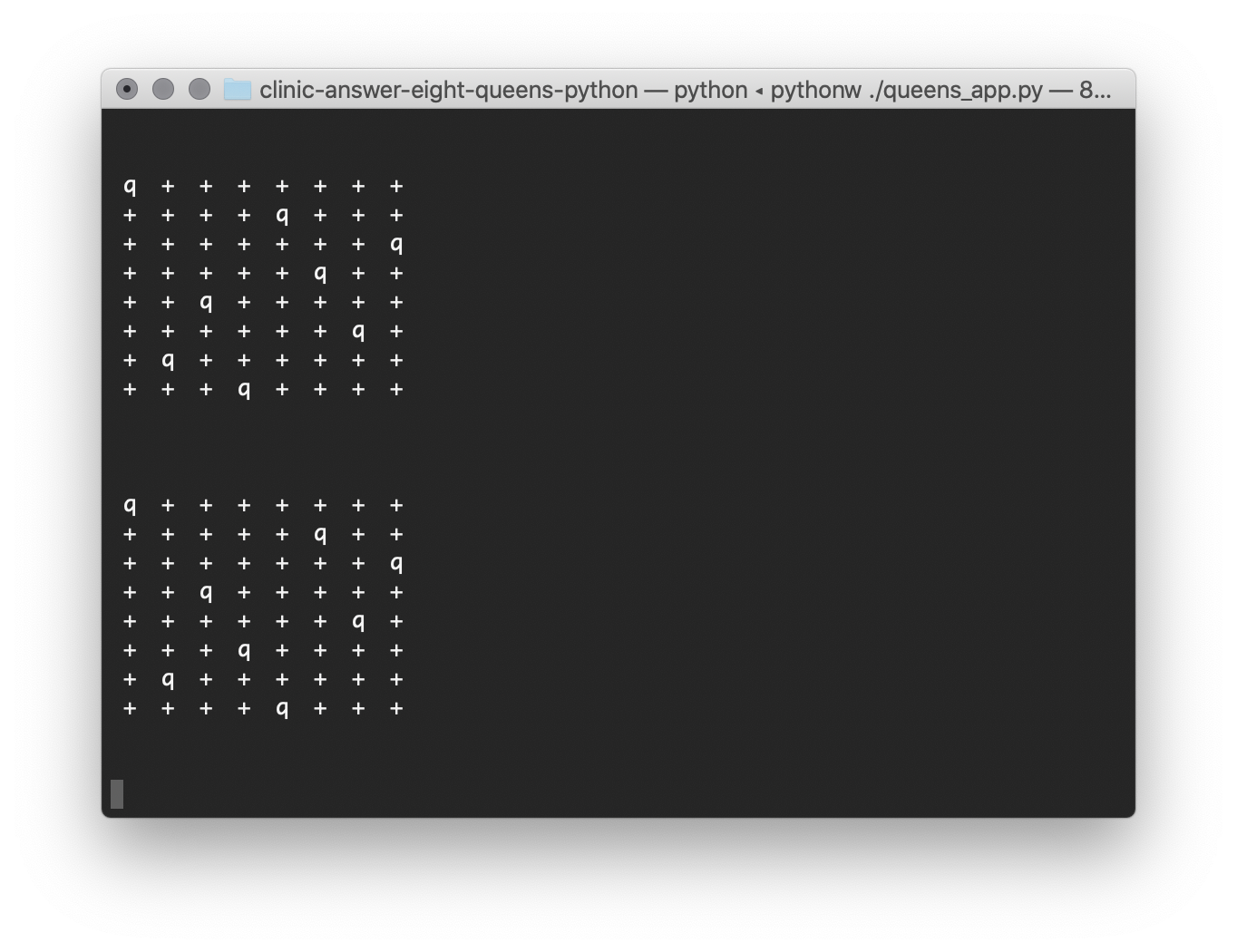### Eight Queens Solver App, Python, on Ubuntu 18.04 LTS.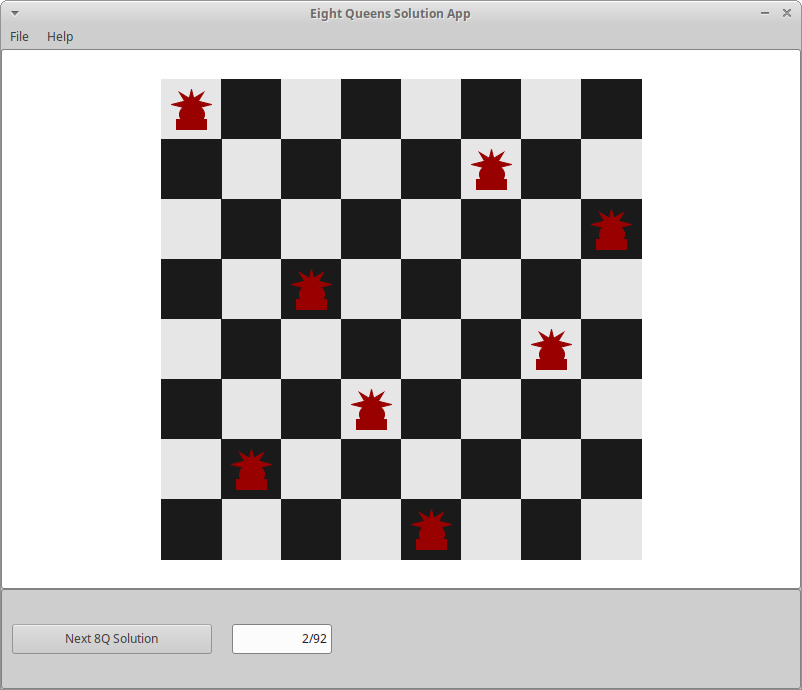### Eight Queens Solver App, Python, on Windows 10 20H2.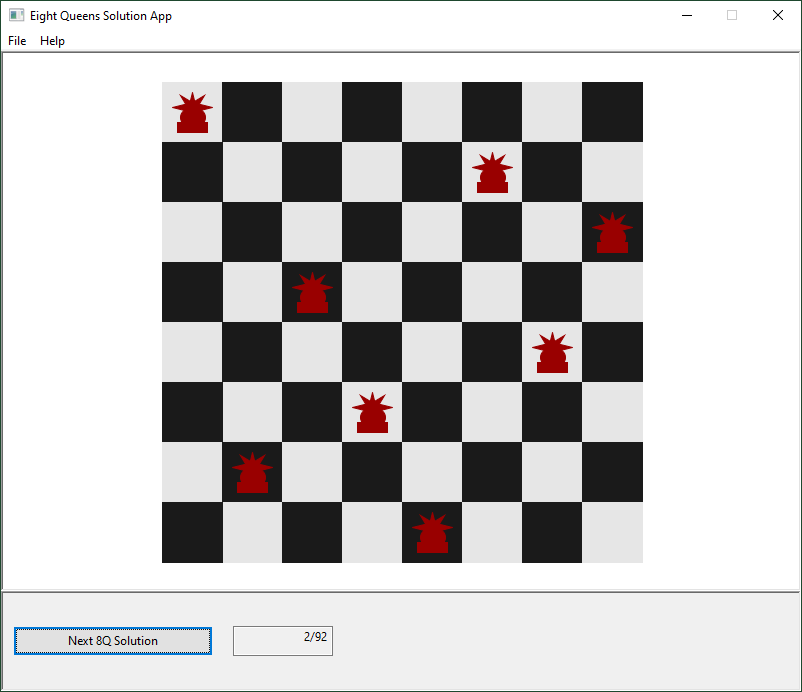## Hints for cross-platform design

### Installing wxPython

``````pip install wxPython
``````

For macOS 10.15 and Windows 10 a Wheel package can be downloaded by `pip` to provide the necessary binaries of wxWidgets 3 to support the wxPython 4.

For Ubuntu Linux, the `pip` command will attempt to compile wxWidgets and wxPython from source. The wxPython 4 wheel may not compile correctly if your system is not configured for from-source compilation. By passing the argument `-v` to `pip install` the compilation process will be visable. `WARNING` remarks from the ./configure script reveal what headers are required to be installed via the Ubuntu `apt-get` package management. This can include the `g++` compiler, as well as headers for `secure`, `libtiff`, `libjpeg`, `gtk 3.0`, `gstreamer 1.0`, `libnotify` and more. On Ubuntu, the command `dpkg-query --list` can be used to view what packages are installed, and give clue as to which headers of which version of an already installed library should be downloaded.

### Running the wxPython 4 application with main loop

Note that the following method of constructing a wx.App and running its MainLoop() call interferes with the Spyder 4 IDE debugging. The command-line debugger can still be invoked by adding the pdb argument to the python invocation: `python3 -m pdb weather_app.py`

``````#!/usr/bin/env pythonw
#
"""This module when called as main instantiates the Eight Queens Solution
Application window and runs."""
import wx
# pylint: disable=no-member

import queens_frame

DEBUG_WX_FRAME = False

class QueensApp(wx.App):
"""This class when instantiated and .MainLoop() called, executes a wxpython
application for interactive eight queens solutions calculations."""

def __init__(self):
"""Instantiate a QueensApp object that controls the execution of this
wxpython application."""
super(QueensApp, self).__init__()
self._frame = None

def OnInit(self): # pylint: disable=invalid-name
"""Called when this wxpython app is initialized and starts main
loop."""
self._frame = queens_frame.QueensFrame(None)
self._frame.Show()
return True

# Executed when the queens_app module is called directly as a script.
if __name__ == "__main__":
app = QueensApp()
if DEBUG_WX_FRAME:
wx.lib.inspection.InspectionTool().Show()
app.MainLoop()
``````

### Running the Python app with Framework support on Ubuntu

``````\$ which python3
/home/timothystotts/anaconda3/bin/python3
\$ python3 queens_app.py
``````

### Running the Python app Framework support on macOS 10.15

``````\$ which pythonw
/Users/timothystotts/opt/anaconda3/bin/pythonw
\$ pythonw queens_app.py
``````

### Running the Python app Framework support on Windows 10

Run the Anaconda3 Powershell Prompt

``````python queens_app.py
``````

## Eight Queens Solver Algorithm implementation

(The execution times were measured with Anaconda 2020.11 Python 3.8 on macOS Catalina 10.15.7 with all updates.)

The chess board was represented as a 8 row list of 9 column lists. The lists contain only Boolean values of True or False. The board is initially populated with one queen per row positioned in column 8 (of zero to eight), representing the queen piece not being placed on the board. From there, a piece is placed at row 0, then row 1, and so forth. Each time a row is placed, the line of sight (rook, bishop, lines of sight) is determined between that row and each of the above rows, the previous row and each of the above rows, back to row 0. A slow and overly computational test of the above rows would be:

``````def test_above(self, above_row):
ret = True

for j in range(above_row, -1, -1): # let be row 2, 1, 0
idx = self._chessboard[j].index(True) # let be col c
for k in range(j - 1, -1, -1): # let be row 1, 0
chkidx = self._chessboard[k].index(True) # let be col b
if (chkidx == idx):
ret = False
elif (abs(chkidx - idx) == abs(j - k)):
ret = False

return ret
``````

With this implementation, the puzzle solver finds 92 correct answers and computes all of them in 0.116s time, compared to the 0.073s of the instructor’s `nqueens.py` implementation. There is an optimization possible here. For each row above which was already successfully placed, that row has already been tested for line-of-sight. Thus, we only need to test the row we are currently placing. The optimization is:

``````def test_above(self, above_row):
ret = True

j = above_row # let be row 2
idx = self._chessboard[j].index(True) # let be col c
for k in range(j - 1, -1, -1): # let be row 1, 0
chkidx = self._chessboard[k].index(True) # let be col b
if (chkidx == idx):
ret = False
elif (abs(chkidx - idx) == abs(j - k)):
ret = False

return ret
``````

With this optimization to the puzzle solver, the solver finds 92 correct answers and computes all of them in 0.044s time. Each row is placed one column at a time; and the solver moves to the next row if the line can be placed by `test_above()` returning `True`; and if the row cannot be placed, it is marked in column 8 as True, and the previous row is tested to be placed at a further down-the-row position; iteratively for the whole board.

One minimal change was made after completing the program. It is arguable that setting a full row to a new value should be done with class constants instead of inline constants. The calculation class now looks like:

``````class QueensCalc:
"""A combined data and behavior definition of a single iteration, or all
iterations, of calculating the Eight Queens solution."""

BOARD_N = 8
ROW_EMPTY = [False, False, False, False, False, False, False, False, False]
ROW_UNPLACED = [False, False, False, False, False, False, False, False, True]

def __init__(self):
``````

and setting a row looks like:

``````self._chessboard[cur_row] = copy.copy(self.ROW_UNPLACED)
``````

instead of:

``````self._chessboard[cur_row] = [False, False, False, False, False, False, False, False, True]
``````

With this style change to the puzzle solver, the solver finds 92 correct answers and computes all of them in 0.048s time. The performance impact was minimal.